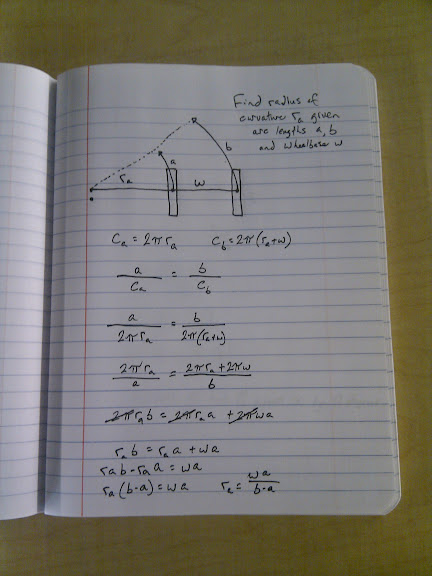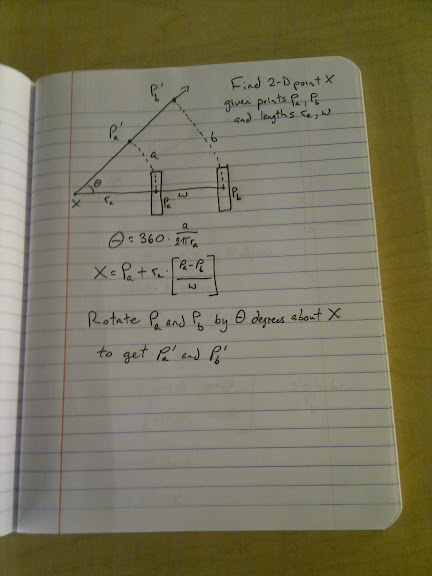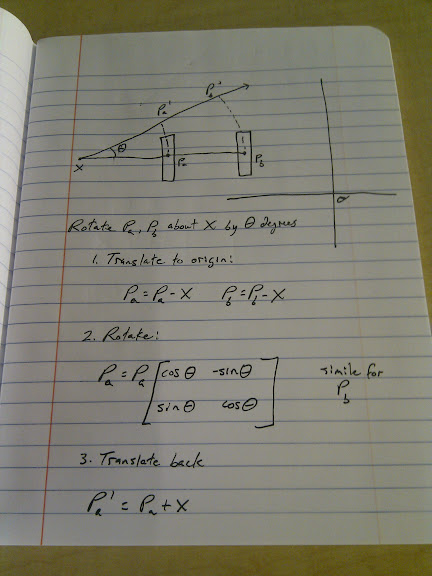## Thursday, June 03, 2010

### Going from odometry to position in a two-wheeled robot

My robot has two drive wheels on which I have odometry (plus some casters that we'll ignore). I want to use the odometry from the wheel encoders to keep track of where I've gone since I started counting.

Let's say the right wheel moves b inches while the left wheel moved a inches during some time interval. Assume b > a. Then if we let the robot keep moving forever with those wheel speeds, the robot will make a big circle counterclockwise on the floor about some point X. We want to find X so that we can use a and b to find the new wheel positions on the floor.We want to find ra, the distance from the left wheel to X.

We start by imagining the circumference of the circle the left wheel would travel in: ca. ca = 2*pi*ra. The right wheel's circle will have a radius of ra+w, where w is the wheelbase of the robot.

Since the two wheels are circling the same point on the floor with the axle always pointing toward the center of the circles, we know the distances a and b will constitute the same proportion of their respective circles. (If a goes all the way around its circle, b must have gone all the way around its circle too. Likewise if a goes 1/10th of the way around, etc.)

So we know that a/ca = b/cb, which is also the proportion of the circle we traveled (if a/ca = 0.10 then we've gone 10% around the circle). Substitute and solve and we get ra = w*a / (b-a).

Great! Now we know our radius of curvature.

Next, we want to find X, the actual point on the floor that we're circling. We start by finding theta, the angle we moved around the circle, by simply multiplying a/ca by 360 (for example, 0.10 * 360 = 36 degrees). If we want it in radians instead of degrees, it's even simpler, since multiplying by 2*PI cancels out the 2*PI in ca, leaving just a/ra.We know that the point X is ra inches to the left of Pa, along a line which also passes through Pb. (X, Pa and Pb are 2-dimensional points). We get a vector from Pb to Pa with (Pa-Pb), make it length 1 by dividing by w, then multiply by ra so it's the right length to reach from Pa to X. Then we add it to Pa so that the vector ends at X.

Now we can find the updated wheel locations on the floor by rotating Pa and Pb by theta degrees around the point X. How do we do that? Well, we translate everything so that X is at the origin, then use a rotation matrix to rotate by theta, then translate back so that X is where it started:Here's code that implements all of that (with saner variable names), plus the case where both wheels move the same amount):

`// Just some math to turn wheel odometry into position updates// Released into the public domain 3 June 2010#include <stdio.h>#include <math.h>#include <stdlib.h>#define PI (3.14159)#define WHEELBASE (12.0)// left wheeldouble Lx = -WHEELBASE/2.0;double Ly = 0.0;// right wheeldouble Rx = WHEELBASE/2.0;double Ry = 0.0;// given distances traveled by each wheel, updates the// wheel position globalsvoid update_wheel_position(double l, double r) {  if (fabs(r - l) < 0.001) {    // If both wheels moved about the same distance, then we get an infinite    // radius of curvature.  This handles that case.    // find forward by rotating the axle between the wheels 90 degrees    double axlex = Rx - Lx;    double axley = Ry - Ly;    double forwardx, forwardy;    forwardx = -axley;    forwardy = axlex;    // normalize    double length = sqrt(forwardx*forwardx + forwardy*forwardy);    forwardx = forwardx / length;    forwardy = forwardy / length;    // move each wheel forward by the amount it moved    Lx = Lx + forwardx * l;    Ly = Ly + forwardy * l;    Rx = Rx + forwardx * r;    Ry = Ry + forwardy * r;        return;  }  double rl; // radius of curvature for left wheel  rl = WHEELBASE * l / (r - l);  printf("Radius of curvature (left wheel): %.2lf\n", rl);  double theta; // angle we moved around the circle, in radians  // theta = 2 * PI * (l / (2 * PI * rl)) simplifies to:  theta = l / rl;  printf("Theta: %.2lf radians\n", theta);  // Find the point P that we're circling  double Px, Py;  Px = Lx + rl*((Lx-Rx)/WHEELBASE);  Py = Ly + rl*((Ly-Ry)/WHEELBASE);  printf("Center of rotation: (%.2lf, %.2lf)\n", Px, Py);  // Translate everything to the origin  double Lx_translated = Lx - Px;  double Ly_translated = Ly - Py;  double Rx_translated = Rx - Px;  double Ry_translated = Ry - Py;  printf("Translated: (%.2lf,%.2lf) (%.2lf,%.2lf)\n",    Lx_translated, Ly_translated,    Rx_translated, Ry_translated);  // Rotate by theta  double cos_theta = cos(theta);  double sin_theta = sin(theta);  printf("cos(theta)=%.2lf sin(theta)=%.2lf\n", cos_theta, sin_theta);  double Lx_rotated = Lx_translated*cos_theta - Ly_translated*sin_theta;  double Ly_rotated = Lx_translated*sin_theta + Ly_translated*sin_theta;  double Rx_rotated = Rx_translated*cos_theta - Ry_translated*sin_theta;  double Ry_rotated = Rx_translated*sin_theta + Ry_translated*sin_theta;  printf("Rotated: (%.2lf,%.2lf) (%.2lf,%.2lf)\n",    Lx_rotated, Ly_rotated,    Rx_rotated, Ry_rotated);  // Translate back  Lx = Lx_rotated + Px;  Ly = Ly_rotated + Py;  Rx = Rx_rotated + Px;  Ry = Ry_rotated + Py;}main(int argc, char **argv) {  if (argc != 3) {    printf("Usage: %s left right\nwhere left and right are distances.\n",      argv);    return 1;  }  double left = atof(argv);  double right = atof(argv);  printf("Old wheel positions: (%lf,%lf) (%lf,%lf)\n",    Lx, Ly, Rx, Ry);  update_wheel_position(left, right);  printf("New wheel positions: (%lf,%lf) (%lf,%lf)\n",    Lx, Ly, Rx, Ry);}`